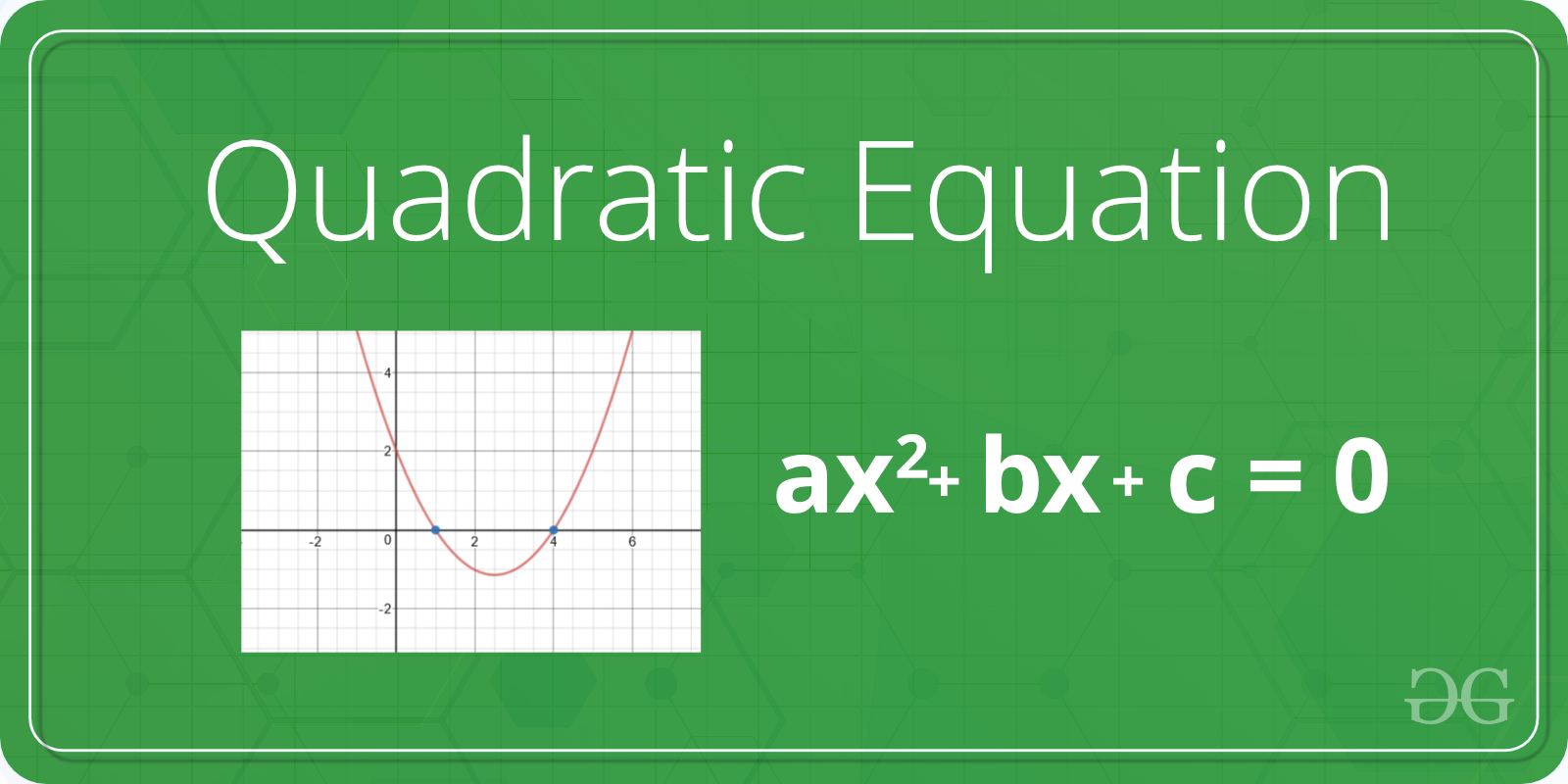# Python program to solve quadratic equation

• Difficulty Level : Basic
• Last Updated : 29 Aug, 2020

Given a quadratic equation the task is solve the equation or find out the roots of the equation. Standard form of quadratic equation is –

ax2 + bx + c
where,
a, b, and c are coefficient and real numbers and also a ≠ 0.
If a is equal to 0 that equation is not valid quadratic equation.Examples:

Input :a = 1, b = 2, c = 1
Output :
Roots are real and same
-1.0

Input :a = 2, b = 2, c = 1
Output :
Roots are complex
-0.5  + i 2.0
-0.5  - i 2.0

Input :a = 1, b = 10, c = -24
Output :
Roots are real and different
2.0
-12.0


Method 1: Using the direct formula

Using the below quadratic formula we can find the root of the quadratic equation.There are following important cases.

If b*b < 4*a*c, then roots are complex
(not real).
For example roots of x2 + x + 1, roots are
-0.5 + i1.73205 and -0.5 - i1.73205

If b*b == 4*a*c, then roots are real
and both roots are same.
For example, roots of x2 - 2x + 1 are 1 and 1

If b*b > 4*a*c, then roots are real
and different.
For example, roots of x2 - 7x - 12 are 3 and 4

 # Python program to find roots of quadratic equationimport math     # function for finding rootsdef equationroots( a, b, c):       # calculating discriminant using formula    dis = b * b - 4 * a * c     sqrt_val = math.sqrt(abs(dis))           # checking condition for discriminant    if dis > 0:         print(" real and different roots ")         print((-b + sqrt_val)/(2 * a))         print((-b - sqrt_val)/(2 * a))           elif dis == 0:         print(" real and same roots")         print(-b / (2 * a))           # when discriminant is less than 0    else:        print("Complex Roots")         print(- b / (2 * a), " + i", sqrt_val)         print(- b / (2 * a), " - i", sqrt_val)   # Driver Program a = 1b = 10c = -24  # If a is 0, then incorrect equationif a == 0:         print("Input correct quadratic equation")   else:    equationroots(a, b, c)

Output:

real and different roots
2.0
-12.0


Method 2: Using the complex math module

First, we have to calculate the discriminant and then find two solution of quadratic equation using cmath module.

 # import complex math moduleimport cmath  a = 1b = 4c = 2  # calculating  the discriminantdis = (b**2) - (4 * a*c)  # find two resultsans1 = (-b-cmath.sqrt(dis))/(2 * a)ans2 = (-b + cmath.sqrt(dis))/(2 * a)  # printing the resultsprint('The roots are')print(ans1)print(ans2)

Output:

The roots are
(-3.414213562373095+0j)
(-0.5857864376269049+0j)


My Personal Notes arrow_drop_up